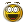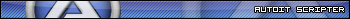Recommended Posts

Hello anybody!

I want to ask if anybody user here that can help me little and can give me some tips how to find a file extension

Example if I have a file:  TESTING.EXE how to find out the exe or .exe for that file what code i must have to find that.

Share on other sites

Use _PathSplit() !
Or my function:

Func _SplitPath(\$sFilePath, \$sType = 0)
Local \$sDrive, \$sDir, \$sFileName, \$sExtension, \$sReturn
Local \$aArray = StringRegExp(\$sFilePath, "^\h*((?:\\\\\?\\)*(\\\\[^\?\/\\]+|[A-Za-z]:)?(.*[\/\\]\h*)?((?:[^\.\/\\]|(?(?=\.[^\/\\]*\.)\.))*)?([^\/\\]*))\$", 1)
If @error Then
ReDim \$aArray
\$aArray = \$sFilePath
EndIf
\$sDrive = \$aArray
If StringLeft(\$aArray, 1) == "/" Then
\$sDir = StringRegExpReplace(\$aArray, "\h*[\/\\]+\h*", "\/")
Else
\$sDir = StringRegExpReplace(\$aArray, "\h*[\/\\]+\h*", "\\")
EndIf
\$aArray = \$sDir
\$sFileName = \$aArray
\$sExtension = \$aArray
If \$sType = 1 Then Return \$sDrive
If \$sType = 2 Then Return \$sDir
If \$sType = 3 Then Return \$sFileName
If \$sType = 4 Then Return \$sExtension
If \$sType = 5 Then Return \$sFileName & \$sExtension
If \$sType = 6 Then Return \$sDrive & \$sDir
If \$sType = 7 Then Return \$sDrive & \$sDir & \$sFileName
Return \$aArray
EndFunc   ;==>_SplitPath

Regards,

Share on other sites

Thanks Trong for this example but I can not have that to work can you explain how you insert a file to this, example: file  TEST.EXE and then

pick upp \$sExtension

Share on other sites

Hello. Just for fun

MsgBox(0, "", _GetExtension("test.test.exe"))
MsgBox(0, "", _GetExtension("test.xml"))
MsgBox(0, "", _GetExtension("test"))
MsgBox(0, "", _GetExtension("test.name.test.temp"))

Func _GetExtension(\$sName)
Return StringMid(\$sName, StringInStr(\$sName, ".", 0, -1))
EndFunc   ;==>_GetExtension

SaludosDanysys.comAutoIt...

Share on other sites
1 hour ago, Borje said:

Thanks Trong for this example but I can not have that to work can you explain how you insert a file to this, example: file  TEST.EXE and then

pick upp \$sExtension

@Danyfirex Trong consider add something like this Global Enum \$eDrive=1, \$eDir, \$eFileName, \$eExtension, \$eFullFileName, \$ePath, \$ePathNoEXT

Global Enum \$eDrive = 1, \$eDir, \$eFileName, \$eExtension, \$eFullFileName, \$ePath, \$ePathNoEXT

Global \$sPathSplit = _SplitPath(@ScriptFullPath)

ConsoleWrite("+ Path IN: " & \$sPathSplit & @CRLF)
ConsoleWrite("- Drive: " & \$sPathSplit & @CRLF)
ConsoleWrite("- Dir: " & \$sPathSplit & @CRLF)
ConsoleWrite("- FileName: " & \$sPathSplit & @CRLF)
ConsoleWrite("- Extension: " & \$sPathSplit & @CRLF)
ConsoleWrite("- FullFileName: " & \$sPathSplit & @CRLF)
ConsoleWrite("- Path: " & \$sPathSplit & @CRLF)
ConsoleWrite("- PathNoEXT: " & \$sPathSplit & @CRLF)

Global \$sPathSplit = @SystemDir & "\cmd.exe"
ConsoleWrite("! Path IN: " & \$sPathSplit & @CRLF)
ConsoleWrite("- Drive: " & _SplitPath(\$sPathSplit, \$eDrive) & @CRLF)
ConsoleWrite("- Dir: " & _SplitPath(\$sPathSplit, \$eDir) & @CRLF)
ConsoleWrite("- FileName: " & _SplitPath(\$sPathSplit, \$eFileName) & @CRLF)
ConsoleWrite("- Extension: " & _SplitPath(\$sPathSplit, \$eExtension) & @CRLF)
ConsoleWrite("- FullFileName: " & _SplitPath(\$sPathSplit, \$eFullFileName) & @CRLF)
ConsoleWrite("- Path: " & _SplitPath(\$sPathSplit, \$ePath) & @CRLF)
ConsoleWrite("- PathNoEXT: " & _SplitPath(\$sPathSplit, \$ePathNoEXT) & @CRLF)

Func _SplitPath(\$sFilePath, \$rType = 0)
Local \$aArray = StringRegExp(\$sFilePath, "^\h*((?:\\\\\?\\)*(\\\\[^\?\/\\]+|[A-Za-z]:)?(.*[\/\\]\h*)?((?:[^\.\/\\]|(?(?=\.[^\/\\]*\.)\.))*)?([^\/\\]*))\$", 1)
Local \$rError = @error
ReDim \$aArray
If \$rError Then \$aArray = \$sFilePath ;  Original path
If StringLeft(\$aArray, 1) == "/" Then
\$aArray = StringRegExpReplace(\$aArray, "\h*[\/\\]+\h*", "\/")
Else
\$aArray = StringRegExpReplace(\$aArray, "\h*[\/\\]+\h*", "\\")
EndIf
\$aArray = \$aArray & \$aArray
\$aArray = \$aArray & \$aArray
\$aArray = \$aArray & \$aArray
If \$rType = 1 Then Return \$aArray ; Drive
If \$rType = 2 Then Return \$aArray ; Dir
If \$rType = 3 Then Return \$aArray ; FileName
If \$rType = 4 Then Return \$aArray ; Extension
If \$rType = 5 Then Return \$aArray ; FullFileName : FileName & \$eExtension
If \$rType = 6 Then Return \$aArray ; Path : Drive & \$eDir
If \$rType = 7 Then Return \$aArray ; PathNoEXT : Drive & Dir & FileName
Return \$aArray ; Array with 8 elements
EndFunc   ;==>_SplitPath

Edited by Trong

Regards,

Share on other sites

Thanks very very much Trong now I have this to work I have much to learn.

Share on other sites

@Trong consider add something like this Global Enum  \$eDrive=1,\$eDir,\$eFileName,\$eExtension,\$eFullFileName,\$ePath,\$ePathNoEXT

SaludosDanysys.comAutoIt...

Share on other sites

Hi Danyfirex

Thank you very much for your example that works perfect your little example is was I need thanks again.

Have a nice day !!

Create an account or sign in to comment

You need to be a member in order to leave a comment

Create an account

Sign up for a new account in our community. It's easy!

Register a new account